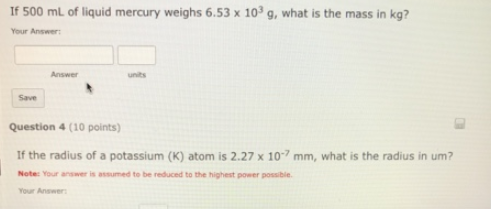# Problem: If 500 mL of liquid mercury weighs 6.53 x 103 g, what is the mass in kg? If the radius of a potassium (K) atom is 2.27 x 10-7 mm, what is the radius in um?

###### FREE Expert Solution
84% (396 ratings)###### Problem Details

If 500 mL of liquid mercury weighs 6.53 x 103 g, what is the mass in kg?

If the radius of a potassium (K) atom is 2.27 x 10-7 mm, what is the radius in um?